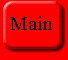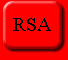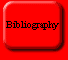## Problems With Public Key

This page is meant to analyze some of the flaws and possible security leaks of public key cryptography. All of the assumptions as to public key systems' security are based both upon the idea that P does not equal NP and thus that there is no polynomial solution to the algorithms, and the hope that there is not some way to solve the problem while avoiding the obvious exhaustive search. This page will present several possibilities for polynomial solutions to the RSA algorithm as well as analyzing the coming of newer and faster hardware.

### Possible Attacks Against RSA

As long as there are people who do not want other people to see what they are sending, there will probably be those who want to get at that information. These people, called attackers, are always searching for some "short-cut" to encryption algorithms so that they do not have to do an exhaustive search which might take months or years. This situation is a lot like a one-way street. Anyone can go in one direction (i.e. encrypt something using a public key), but people cannot decrypt something unless they possess the private key. People could continue on the street in the proper direction and wrap around the world (which is not unlike doing an exhaustive search), but that would almost never be productive. Attackers instead try to find a hidden street that runs parallel to the main street so that they can get to the message without having the key and without spending an inordinate amount of time trying to determine it.

The RSA algorithm is based on the premise that factoring is an NP complete problem. In other words, it is thought that to find the factors of a number, the only way to do so is to try every number that is less than the square root of the number. There are two possibilities that would lead to a break in the RSA algorithm: if factoring was found to be calculable in polynomial time or if an attacker could somehow find a way to avoid doing an exhaustive search of possible factors. Since no one has found a way to factor in polynomial time, attackers are left looking for some other way to avoid doing an exhaustive search, just like Adi Shamir did in his "break" of the knapsack problem.

One possible attack on RSA plays on a characteristic of the RSA algorithm; that it takes the algorithm different amounts of time to decrypt different inputs when using the same key. Using this fact, if an attacker can passively eavesdrop on an interactive protocol and record the time it takes the victim to process different cryptotexts, he can gain some insight as to the key. In the equation used to decrypt cryptotext, m = c^d mod n, where m is the original message, c is the encrypted text, n is the public key, and d is the private key, c can be intercepted, n is known to the public, and the attacker wishes to calculate d so that s/he can decrypt c into m. If the attacker can time the victim while s/he computes c^d mod n for several values of c using the same d, then the attacker can calculate d and get m. And s/he can do so only having to do 4 times as many operations as the private key holder would have to do.

The attack focuses on finding the private key bit by bit. If someone knows exponent bits 0..(b-1) they can determine bit b. To find out the entire exponent, one must just start with b=0 and repeat until the entire key is known. As the attacker progresses down the exponent bit, if the next bit b is 1 then mb = (sb * c) mod n will be computed, if it is zero the operation will be skipped. The attacker first analyzes a system that uses a modular multiplication function that is usually very fast but every so often takes a lot more time than an entire normal modular exponentiation. For some values of sb the calculation of mb = (sb * c) mod n will be very slow (the attacker can determine which these are by using knowledge about the system's design). If the total modular exponentiation time is ever fast when mb = (sb * y) mod n should be slow, the attacker knows that exponent bit b must be zero. If, however, the operations are always done slowly, then exponent bit b is probably 1.

### Hardware of the future

Perhaps one might assume that larger, faster computers will enable "hackers" to break codes more easily and thus make public key cryptosystems less secure. This is not the case, however, since this newer technology also lends itself to picking and using larger keys and better algorithms. In fact, new hardware will benefit legal encryptors far more than it will aid "code breakers". There is, however, one technological prospect that offers a strong challenge to the RSA and many other such algorithms: quantum computers. While still theoretical, should quantum computers be able to be produced they would be able to solve such algorithms as the RSA very quickly.

Traditional computers operate on the normal bit which can have one of two states (0 or 1). Quantum computers, on the other hand, operate on what are called quantum bits or qubits. A qubit is merely a linear superposition of the two states (0 and 1). A quantum register is thus made out of some number n qubits. The reason that quantum computers are able to solve what are traditionally thought of as exponential problems in polynomial time is a concept known as quantum parallelism, where the computer is able to work exponentially. This is not unlike the idea of a replicating Turing machine, where when it gets to a certain point, it can branch off into multiple states. Once quantum computers can be realized, they will be able to compute discrete logarithms in polynomial time. The reason that they are still not here is due to something called quantum decoherence which is due to the influence of the outside environment.